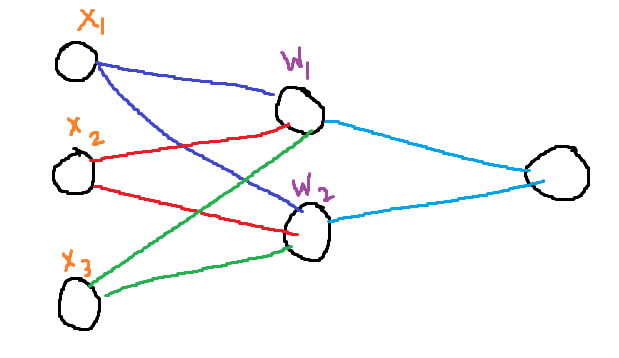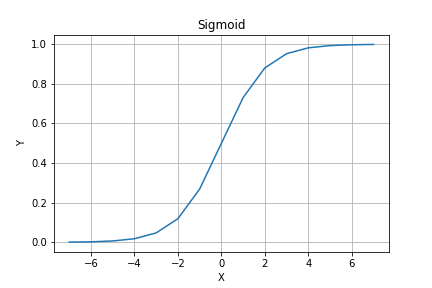# All the ways to initialize your neural networkIn this article, I evaluate the many ways of weight initialization and current best practices.

## Zero Initialization

*Initializing weights to zero DOES NOT WORK. *Then Why have I mentioned it here? To understand the need for weight initialization, we need to understand why initializing weights to zero *WON’T *work.

Let us consider a simple network like the one shown above. Each input is just one scaler X₁, X₂, X₃. And the weights of each neuron are W₁ and W₂. Each weight update is as below:

Out₁ = X₁W₁ + X₂W₁ + X₃*W₁

Out₂ = X₁W₂ + X₂W₂ + X₃*W₂

As you can see by now, if the weight matrix W = [W₁ W₂] is initialized to zero, then both out1 and out2 are exactly the same.

Even if we add a non zero random bias term to both, The weights are updated to non-zero, However, they will remain identical, hence both neurons of the hidden unit are computing the same thing. In other words, they are Symmetrical.

This is highly undesirable as this is wasted computation. This is why zero initialization does not work.

## Random Initialization

Now that we know the weights have to be different, the next idea was to initialize these weights randomly. Random initialization is much better than zero initialization, but can these random numbers be _ANY _number?

Let’s assume you are using sigmoid non-linearity. The function is drawn below.Sigmoid. Image by the author.

We can see that even for values as big as 6 the value of sigmoid is almost 1, and for values as small as -6 the value of sigmoid is zero. This means if our weight matrix is initialized to values that are either too big or too small, all useful information is lost in the sigmoid function.

This is not as important if we use a ReLu non-linearity, but there are other problems with weights being initialized to large or small values. There are better ways to initialize our weights.

## Weight Initialization in Deep Neural Networks

Learn about different weight initialization methods in neural networks. In this article, I will first explain the importance of the wight initialization and then discuss the different methods that can be used for this purpose.

## Weight Initialization for Neural Networks — Does it matter?

Weight Initialization for Neural Networks — Does it matter? A comparative study of different weight initialization techniques

## Weight Initialization for Neural Networks — Does it matter?

Weight Initialization for Neural Networks — Does it matter? A comparative study of different weight initialization techniques

## Why Deep Learning Ensembles Outperform Bayesian Neural Networks

Don’t they do the same thing? Why Deep Learning Ensembles Outperform Bayesian Neural Networks

## Cheat Sheets for AI, Neural Networks, Machine Learning, Deep Learning & Big Data

Cheat Sheets for AI, Neural Networks, Machine Learning, Deep Learning & Big Data